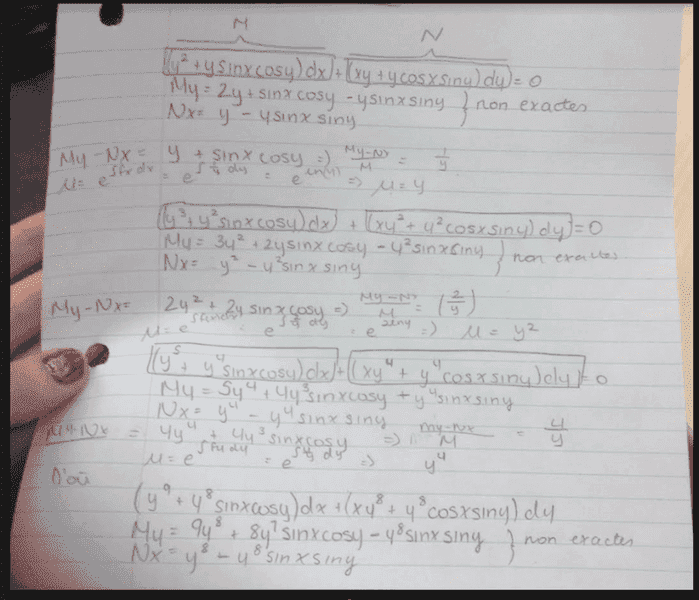# Initial value problem - differential equations

• Cocoleia
In summary, the conversation discusses solving a given equation with the initial condition of y = π/2, and the attempt to make the equation exact. After dividing both sides by y, it is found that the equation is not always possible to make exact, as it may involve dividing by zero. The final answer is xy - cos(x)cos(y) = 0.

## Homework Statement

I am given (y^2 + y sin x cos y) dx + (xy + y cos x sin y) dy = 0, y(0) = π/2 .
I need to solve this

## The Attempt at a SolutionAt this point they still aren't exact, so I gave up. I can't figure out what the problem is. Is it possible that I have to continue trying to make them exact?

I know that the answer is: xy-cosxcosy=0

Cocoleia said:

## Homework Statement

I am given (y^2 + y sin x cos y) dx + (xy + y cos x sin y) dy = 0, y(0) = π/2 .
I need to solve this

## The Attempt at a Solution

View attachment 110019

At this point they still aren't exact, so I gave up. I can't figure out what the problem is. Is it possible that I have to continue trying to make them exact?

I know that the answer is: xy-cosxcosy=0
Divide both sides by y, to get ##(y + \sin(x)\cos(y))dx + (x + \cos(x)\sin(y)) dy = 0##.
Now check for exactness. Note that since ##y(0) = \pi/2##, it's reasonable to assume that ##y \ne 0##.

Mark44 said:
Divide both sides by y, to get ##(y + \sin(x)\cos(y))dx + (x + \cos(x)\sin(y)) dy = 0##.
Now check for exactness. Note that since ##y(0) = \pi/2##, it's reasonable to assume that ##y \ne 0##.
Can we always do this if we have a common factor like y in this case?
Thank you, I spent a very long time trying to figure this out

Cocoleia said:
Can we always do this if we have a common factor like y in this case?
I wouldn't say you can always do it. By getting rid of a factor of y, we are possibly dividing by zero. Since the initial condition is that ##y(0) = \pi/2##, if necessary, we can restrict y to some interval that includes ##\pi/2## but doesn't include zero.